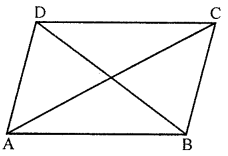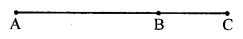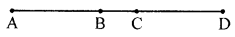## ML Aggarwal Class 6 Solutions for ICSE Maths Chapter 11 Understanding Symmetrical Shapes Ex 11.1

Question 1.
Why is it better to use a divider and a ruler than a ruler only, while measuring the length of a line segment?
Solution:
There may be errors due to the thickness of the ruler and angular viewing by using a ruler. These errors are eradicated by using a divider. So, it is better to use a divider with ruler, than a ruler only, while measuring the length of a line segment.

Question 2.
In the given figure, compare the line segments with the help of a divider and fill in the blanks by using the symbol >, = or <:
(i) AB ………. CD
(ii) BC ………. AB
(iii) AC ………. BD
(iv) CD ………. BDSolution:
(i) AB = CD
(ii) BC < AB
(iii) AC > BD
(iv) CD < BD

Question 3.
If A, B and C are collinear points such that AB = 6 cm, BC = 4 cm and AC = 10 cm, which one of them lies between the other two?
Solution:
Given : A, B and C are colinear pointsPoint B lies between A and C.

Question 4.
In the given figure, verify the following by measurement:
(i) AB + BC = AC
(ii) AC – BC = ABSolution:
Measure with help of divider and ruler.
AB = 3 cm, BC = 1.5 cm and AC = 4.5 cm
(i) AB + BC = AC
⇒ 3 cm + 1.5 cm = 4.5 cm
⇒ 4.5 cm = 4.5 cm
(ii) AC – BC = AB
⇒ 4.5 cm – 5 cm = 3 cm
⇒ 3 cm = 3 cm

Question 5.
In the given figure, verify by measurement that:
(i) AC + BD = AD + BC
(ii) AB + CD = AD – BCSolution:
Measure with help of divide and ruler.
AB = 1.8 cm, BC = 0.8 cm, BD = 2.7 cm CD = 1.9 cm, AC = 2.6 cm and AD = 4.5 cm
(i) AC + BD = AD + BC
⇒ 2.6 cm + 2.7 cm = 4.5 cm + 0.8 cm
⇒ 5.3 cm = 5.3 cm
(ii) AB + CD = AD – BC
⇒ 1.8 cm = 1.9 cm = 4.5 cm – 0.8 cm
⇒ 3.7 cm = 3.7 cm

Question 6.
In the given figure, measure the lengths of the sides of the triangle ABC and verify:
(i) AB + BC > AC
(ii) BC + AC > AB
(iii) AC + AB > BCSolution:
Measure with help of divide and ruler.
AB = 2.5 cm, BC = 3.8 cm, AC = 4 cm
(i) AB + BC > AC
⇒ 2.5 + 3.8 cm > 4 cm
⇒ 5.3 cm > 4 cm
(ii) BC + AO > AB
⇒ 3.8 + 4 cm > 2.5 cm
⇒ 7.8 cm > 7.5 cm
(iii) AC + AB > BC
⇒ 4 cm + 2.5 cm > 3.8 cm
⇒ 6.5 cm > 3.8 cm